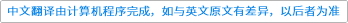Bulletin des Sciences Mathématiques ( IF 1.241 ) Pub Date : 2021-06-07 , DOI: 10.1016/j.bulsci.2021.103012
Thomas Kalmes

For a constant coefficient partial differential operator $P\left(D\right)$ with a single characteristic direction, such as the time-dependent free Schrödinger operator as well as non-degenerate parabolic differential operators like the heat operator, we characterize when open subsets ${X}_{1}\subseteq {X}_{2}$ of ${\mathbb{R}}^{d}$ form a P-Runge pair. The presented condition does not require any kind of regularity of the boundaries of ${X}_{1}$ nor ${X}_{2}$. As part of our result we prove that for a large class of non-elliptic operators $P\left(D\right)$ there are smooth solutions u of the equation $P\left(D\right)u=0$ on ${\mathbb{R}}^{d}$ with support contained in an arbitarily narrow slab bounded by two parallel characteristic hyperplanes for $P\left(D\right)$.down
wechat
bug